International Journal of Computational and Theoretical Chemistry
Volume 3, Issue 3, May 2015, Pages: 19-27

Effect of Different Shape of Periodic Forces on Chaotic Oscillation in Brusselator Chemical System

Guruparan S.1, Ravindran Durai Nayagam B.2, Jeyakumari S.3, Chinnathambi V.3, *

1Department of Chemistry, Sri K. G. S Arts College, Srivaikuntam, Tamilnadu, India

2Department of Chemistry, Pope’s College, Sawyerpuram, Tamilnadu, India

3Department of Physics, Sri K. G. S Arts College, Srivaikuntam, Tamilnadu, India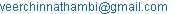(Chinnathambi V.)

Guruparan S., Ravindran Durai Nayagam B., Jeyakumari S., Chinnathambi V.. Effect of Different Shape of Periodic Forces on Chaotic Oscillation in Brusselator Chemical System. International Journal of Computational and Theoretical Chemistry. Vol. 3, No. 3, 2015, pp. 19-27. doi: 10.11648/j.ijctc.20150303.11

Abstract: Bifurcations of periodic orbits and chaos in the Brusselator chemical reaction with different shape of external periodic forces are studied numerically. The external periodic forces considered are sine wave, square-wave, rectified sine wave, symmetric saw-tooth wave, asymmetric saw-tooth wave and modulus of sine wave. Period doubling bifurcations, chaos, reverse period doubling bifurcations, quasiperiodic bifurcations are found to occur due to the applied forces. Parametric regimes where suppression of chaos occurs are predicted. Numerical investigations including bifurcation diagram, maximal Lyapunov exponent, Poincare map, Phase portrait and time series plot are used to detect chaos, quasiperiodic and periodic orbits.

Keywords: Brusselator, Various Periodic Forces, Bifurcations, Chaos, Quasiperiodic Orbit

1. Introduction

The study of nonlinear chemical dynamics (NCD) has flourished in the past three decades [1-10]. The prototypical phenomenon of NCD is chemical oscillation. Complex dynamical behaviours such as periodic, quasiperiodic and chaotic oscillations have been observed in certain nonlinear chemical systems. For example, in Belousov-Zhabotinsky (BZ) reaction, Roux  and Swinney [12,13] and co-workers revealed a class period doubling sequence leading to chaotic behaviour and Hudson and co-workers [14,15] found chaotic behaviour interspersed in sequences of mixed-mode oscillations. Another oscillation reaction known as Peroxidase - Oxidase (PO) has been extensively investigated over the years. In the PO reaction, Yamazaki et al.  observed damped oscillations and Nakamura et al.  found sustained oscillations. Recent studies of the PO reaction have identified not only a period doubling route to chaos but also unstable periodic orbits in the chaotic attractor of the system [18-20]. Quasiperiodicity has also been found . Other systems exhibiting chaotic behaviour include the chlorite–thiosulfate reaction  and the cobalt / manganese / bromide–catalyzed autoxidations of p-xylene and cyclohexanone . Although chaos is usually studied in open systems, several recent investigations have demonstrated that transient chaos may occur in closed systems [24,25], where the changing composition of the system as reactants are consumed serves as the bifurcation parameter.

Complex oscillations and chaos have also been studied in many types of electrochemical processes. Many of the studies of electrochemical chaos have utilized metal electro dissolution reaction. For example, Schell and Albahadily  studied the electro dissolution of copper in H3PO4 in which simple period doubling as well as period doubling mixed-mode oscillations was found. A series of investigations of the electro dissolution of copper in NaCl and H2SO4 carried out by Hudson and Bassett [27-29] in which a number of routes to chaos such as period doubling, quasiperiodicity, intermittency and Shil’nikov chaos were demonstrated. Studies of the electro catalytic oxidations of formaldehyde, formic acid and various alcohols have also yielded information about mixed-mode, quasiperiodic and chaotic behaviours [30,31]. Experimental studies by Koper et al. [32,33] have characterized the mixed-mode and chaotic oscillations found in the system such as the reduction of an In (III) at a hanging mercury electrode in solutions containing thiocyanate ion.

In the past few years much attention has been focused on the study of transition from periodic to chaotic motion in many dynamical systems with external force being of the form f sinωt [3-5,34,35]. Recently many studies have shown that the effect of different kinds of periodic forcing on these systems is considerable and they can change the dynamical behaviours drastically. For example, onset of homoclinic chaos by a periodic string of pulses modulated by Jacobian elliptic function and periodic δ-function , generation of chaotic behaviour by a distorted force , anti-control of chaos by certain periodic forces , suppression of chaos by δ-pulse , stochastic resonance [40,41], nonescape dynamics  and the occurrence of horseshoe chaos  with different form of periodic forces have been reported.

In this paper, we numerically study the effect of different shape of periodic forces on chaotic oscillation in Brusselator chemical system. The text of the paper is organized as follows. In section II, we analyzed the mathematical model, fixed point and stability analysis of the Brusselator chemical system. The mathematical form of various periodic forces considered in our present work is presented in section III. We analyzed the bifurcation structures due to the applied periodic forces such as sine wave, square-wave, rectified sine wave, symmetric saw-tooth wave, asymmetric saw-tooth wave and modulus of sine wave in section IV. For a particular set of values of the parameters of the system, we numerically integrated the system separately for each periodic force we show the occurrence of various routes to chaos, crises and chaos. Finally section V contains the conclusion of the present work.

2. Forced Brusselator Chemical System

The Brusselator is one of the simplest models in nonlinear chemical systems. It has six components, four of which retain constants and the other two permit their concentrations vary with time and space. The classical Brusselator chemical reaction consists of four irreversible steps, given by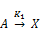B+X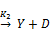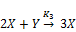(1)

X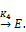Where A and B are constant components, D and E are products and X and Y are the two components variable in time and space. The dynamics of the Brusselator chemical reaction can be described by a system of two ordinary differential equations (ODE’s). In dimensionless form, they are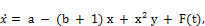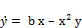(2)

where a and b are constants with a, b > 0, x and y represent the dimensionless concentrations of two reactants. F(t) is the time dependent external periodic driving force. Over the years, there have been extensive studies in the Brusselator system (Eq.2) [44-48] and the references there in. Recently, Ali Sanayei  studied the effect of sinusoidal force in the Brusselator chemical reaction. The unperturbed system (ie F(t) =0 ) has only one equilibrium point (x* , y* ) = (a , b/a) . From the linear stability analysis, the eigenvalues of the fixed point (a , b/a) are obtained as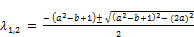(3)

If b > (a2+1) then the eigenvalues of the fixed point λ1 and λ2 are complex conjugate with real part greater than zero and hence is a unstable. If b < (a2+1) the corresponding eigenvalues λ1 and λ2 are complex conjugate with the real part less than zero and hence is a stable. For b > (a2+1), a limit cycle behaviour occurs and is shown in Fig. 1(a). At b = (a2+1), the eigenvalues λ1 and λ2 of the fixed points are purely imaginary that is, λ1,2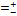ia and hence is a center or elliptic. We have observed Andronov – Hopf bifurcation at b=a2 +1 which is shown in Fig. 1(b).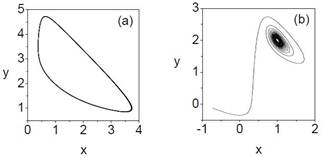Fig. 1. Phase portraits of the unperturbed Brusselator system (ie. F(t) = 0) (a) limit cycle behaviour at b > a2 +1 with a = 1 and b = 3 (b) Andronov – Hopf bifurcation at b = a2 +1 with a = 1 and b = 2.

3. Types of Periodic Forces

Figure 2 depicts various periodic forces considered in our present work. The period-T of all the external periodic forces considered in our study is fixed as 2π/ω, where ω is the angular frequency of the forces. The mathematical form of the periodic forces such as sine wave, square-wave, rectified sine wave, symmetric saw-tooth wave, asymmetric saw-tooth wave and modulus of sine wave are given by,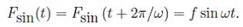(4)(5)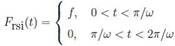(6)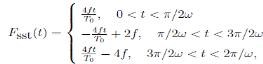(7)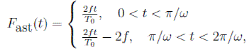(8)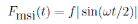(9)

Where T0 = 2π/ω and t is taken as mod (T0).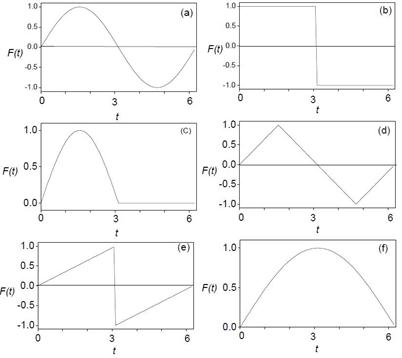Fig. 2. Shape of various periodic forces (a) sine wave (b) square-wave (c) rectified sine wave (d) symmetric saw-tooth wave (e) asymmetric saw-tooth wave (f) modulus of sine wave. For all the forces period is 2π/ω , ω = 1 and amplitude f is 1.

4. Analysis of Bifurcation Structures Due to the Applied Periodic Forces

In this section, we analyze the bifurcation structures due to the different shape of periodic forces applied on the Brusselator system (Eq.2). For our numerical study we fix the parameters values as a = 0.4, b = 1.2 and ω = 0.81. Eq. (2) is solved with different periodic forces by fourth order Runge-Kutta method with time step size (2π/ω)  / 200. Numerical solution corresponding to first 500 drive cycle is left as transient. We analyzed the behavior of the Brusselator system (Eq. 2) by varying the forcing amplitudes of each periodic force. Figure 3 shows the bifurcation diagrams and the corresponding maximal Lyapunov exponent (λm) diagrams for various periodic forces. The maximal Lyapunov exponent (λm) is computed using the algorithm given in Ref. . If λmax < 0 , the disturbed trajectory is attracted eventually to a stable periodic orbit. λmax > 0 reveals an unstable and chaotic trajectory and λmax = 0 means that the disturbed oscillation and the original oscillation stay apart be a constant mean distance for an indefinite duration until perturbed again. When the forcing amplitude f is varied we found many similarities and differences in the bifurcation patterns which is clearly seen in Fig. 3. The magnification of a part of bifurcation diagrams are shown in Fig. 4. Consider the effect of the force f sin ωt. Figure 4(a) shows the bifurcation diagram of Brusselator system (Eq. 2) driven by sine wave force. As f is increased from zero, a quasiperiodic orbit occurs, which persists up to f = 0.00955 and then it loses its stability giving birth to a period doubling orbit. Brusselator system (Eq.2) then undergoes further period doubling bifurcations as the control parameter f is smoothly varied. For example at f = 0.03345, the period-2T orbit becomes unstable and gives birth to period-4T orbit. This cascade of bifurcation continues further as 8T, 16T,…, orbits and accumulates at f = fc = 0.04578. At this critical value of f onset of chaotic motion occurs. When the parameter f is further increased from fc one finds that chaotic orbits persist for a range of f values followed by reverse period doubling bifurcation. At f = 0.481, the reverse period doubling bifurcation disappears and the long time motion settles to a periodic behaviour. That is the application of sine wave force in Brusselator system (Eq. 2) can be used to suppress chaotic motion by choosing its value in the interval 0.481 < f < 1.0.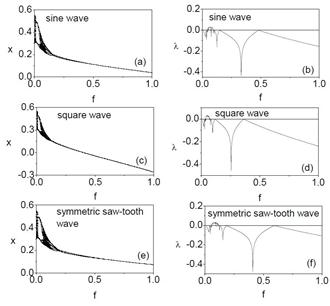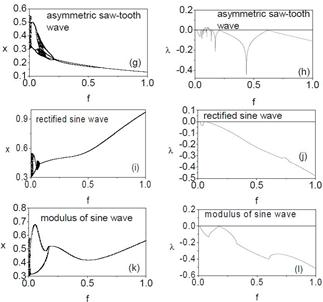Fig. 3. Bifurcation diagrams and the corresponding maximal Lyapunov diagrams of Brusselator system (Eq.2) driven by various periodic forces. The other parameters values are a = 0.4, b = 1.2 and ω = 0.81.

When the force f sin ωt is replaced by other forces similar behaviour is found to occur, except rectified sine wave and modulus of sine wave forces. In rectified sine wave force, bifurcation from quasiperiodic orbit to reverse period-2T and then again quasiperiodic orbit to period-T is found. But in modulus of sine wave force, bifurcation from quasiperiodic orbit to reverse period-2T orbit and then to period-T orbit is found. Chaotic motion is not observed both in rectified sine wave and modulus of sine wave forces. However these bifurcations occurred at different values of forcing amplitude. The range of values of f at which various bifurcations occur for different forms of forces are summarized in table I. From table I and Fig. 1, we note that when f is increased from a small value period-T orbit is realized much earlier for rectified sine wave while it is realized relatively at a higher value of f for the asymmetric saw-tooth wave.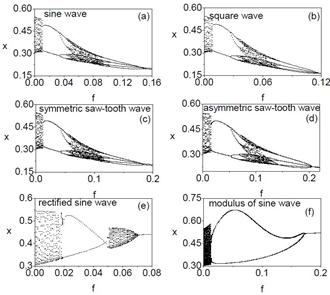Fig. 4. Magnification of a part of bifurcation diagrams in Fig.3.

The bifurcation structures for sine, square, symmetric and asymmetric saw-tooth waves are similar. The bifurcation patterns for the modulus of sine and rectified sine waves are different from others. To understand the observed difference we studied the phase portrait and the corresponding Poincare map by varying the forcing amplitude of all the forces. In all the forces, for small values of f quasiperiodic orbit occurs. Figures 5 and 6 show the phase portraits and the corresponding Poincare maps at f = 0.01 for all forces. From these figures we can clearly confirm the occurrence of quasiperiodic orbit in Brusselator system (Eq. 2). Figures 7 and 8 show the phase portraits and the corresponding Poincare maps at the chaotic orbit for all forces except rectified and modulus of sine wave forces. In these two forces no chaotic orbit is observed which is clearly seen Figs. 4 (e) and (f). Examples of time evolution of the system (Eq. 2) driven by square-wave force with different f values are shown in Fig. 9. The Brusselator system (Eq. 2) exhibits qualitatively different behavior with periodic oscillations in Figs. 9 (a), 9(b), 9(d) and chaos in Fig. 9 (c). For all the forces, from a certain f values, the long time motion settles to a periodic behaviour. For f = 1.0, the motion of the system is periodic with period-T. Figure 10 shows the phase portraits at f = 1.0 for various periodic forces. Cross-well period-T orbit is found for sine wave, square- wave and symmetric saw-tooth wave forces. Cross-well behavior is not found in asymmetric saw-tooth wave, rectified sine wave and modulus of sine wave forces. The geometrical structures of the attractor is different for different forces.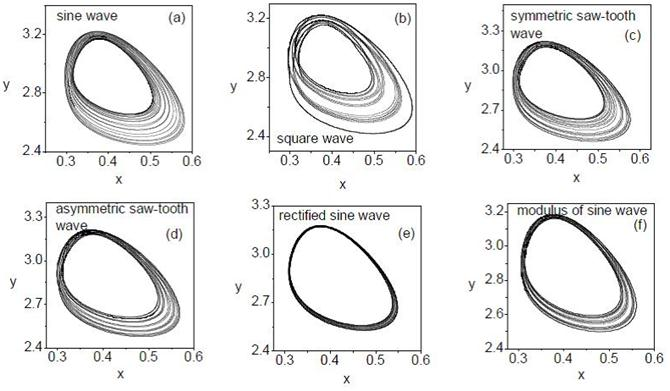Fig. 5. Phase portraits of perturbed Brusselator system (Eq.2) for different periodic forces at f = 0.01. The other parameters values are a = 0.4, b = 1.2 and ω = 0.81.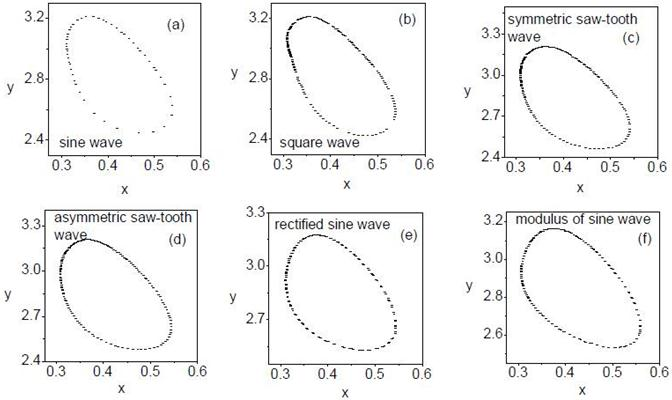Fig. 6. Poincare maps of perturbed Brusselator system (Eq.2) for different periodic forces at f = 0.01. The corresponding parameters are the same as those in Fig.5.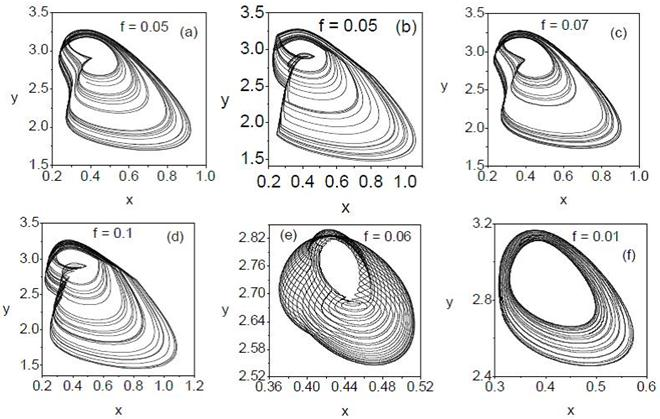Fig. 7. Phase portraits of the perturbed Brusselator system (Eq.2) for different shape of periodic forces such as (a) sine wave (b) square-wave (c) symmetric saw-tooth wave (d) asymmetric saw-tooth wave (e) rectified sine wave (f) modulus of sine wave. The other parameters values are a = 0.4, b = 1.2 and ω = 0.81.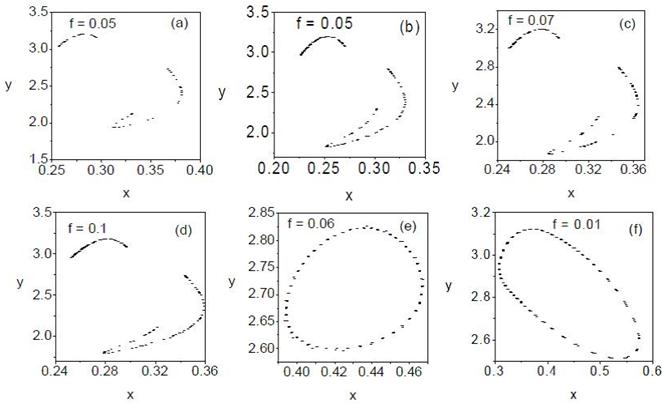Fig. 8. Poincare maps of the perturbed Brusselator system (Eq.2) for different shape of periodic forces such as (a) sine wave (b) square-wave (c) symmetric saw-tooth wave (d) asymmetric saw-tooth wave (e) rectified sine wave (f) modulus of sine wave. The corresponding parameters are the same as those in Fig.7.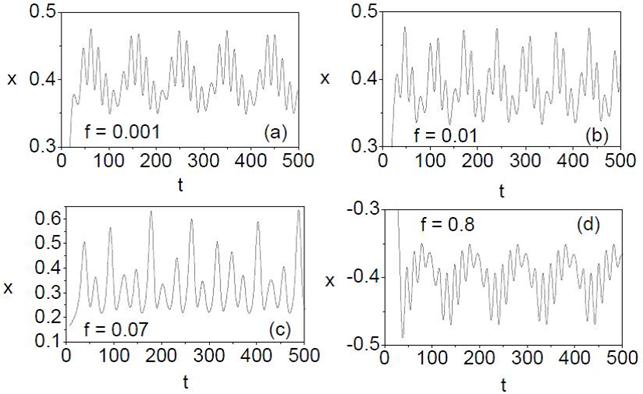Fig. 9. Time evolution of the perturbed Brusselator system (Eq.2) driven by square- wave force (a) quasiperiodic orbit (b) periodic orbit (c) chaotic orbit and (d) reverse periodic orbit. The values of the parameters are a = 0.4, b = 1.2 and ω = 0.81.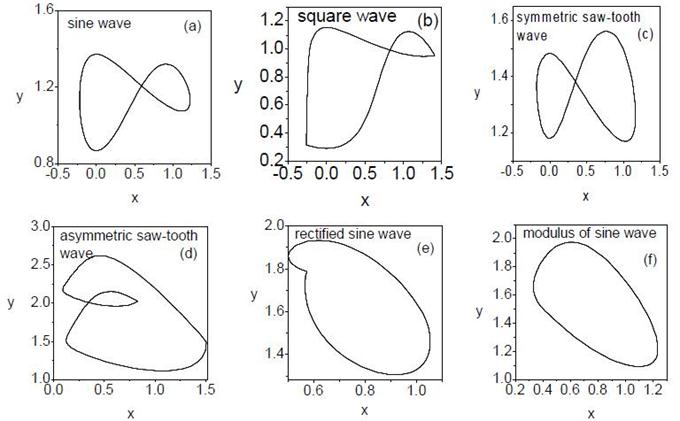Fig. 10. Phase portraits of perturbed Brusselator system (Eq. 2) for different periodic forces at f = 1.0. The values of the other parameters are a = 0.4, b = 1.2 and ω = 0.81.

Table I. Summary of bifurcation phenomena of the Brusselator system (Eq.2) in the presence of different shape of periodic forces for a = 0.4, b = 1.2 and ω = 0.81.

 Types of forces Value of f Nature of solution Sine wave 0 < f < 0.00955 Quasiperiodic orbit 0.00955 Period doublings 0.04578 Chaos 0.09514 < f < 0.481 Reverse period doublings 0.481< f < 1.0 Period-T orbit Square wave 0 < f < 0.00512 Quasiperiodic orbit 0.00512 < f <0.03751 Period doublings 0.03751 Chaos 0.08655 < f<0.36476 Reverse period doublings 0.36476 < f< 1.0 Period-T orbit Symmetric saw-tooth wave 0 < f < 0.01213 Quasiperiodic orbit 0.01213 < f <0.05552 Period doublings 0.05552 Chaos 0.11721 < f<0.59284 Reverse period doublings 0.59284 < f< 1.0 Period-T orbit Asymmetric saw-tooth wave 0 < f < 0.01357 Quasiperiodic orbit 0.01357 < f <0.0726 Period doublings 0.07526 Chaos 0.12373 < f<0.63813 Reverse period doublings 0.63813 < f < 1.0 Period-T orbit Rectified sine wave 0 < f < 0.01622 Quasi periodic orbit 0.01622 Period-2T orbit 0.05021 < f<0.06434 Quasi periodic orbit 0.06434 < f < 1.0 Period-T orbit Modulus of sine wave 0 < f < 0.01423 Quasiperiodic orbit 0.01423< f < 0.17458 Period-2T orbit 0.17458 < f < 1.0 Period-T orbit

5. Conclusion

In this paper we numerically studied the effect of various periodic forces in the Brusselator chemical system (Eq.2). In our present work, we fixed the period of the forces as 2π/ω and ω = 0.81. For a particular set of parameters values, we have shown the occurrence of periodic, quasiperiodic and chaotic oscillations and noticed many similarities and differences in the bifurcation structures in the presence of various periodic forces. In addition, suppression and enhancement of chaos has been found for range of amplitude f of the applied periodic forces.

It is important to study the effect of other types of forces such as amplitude and frequency modulated forces both analytically and numerically in Eq. (2) to investigate certain nonlinear phenomena including resonance, vibrational resonance, ghost vibrational resonance, stochastic resonance etc. These will be studied in future.

References

1. R. J. Field and M. Burger, Oscillations and Travelling waves in Chemical Systems, Wiley- Interscience, New York, 1985.
2. P. Gray and S.K. Scott, Chemical Oscillations, Instabilities: Nonlinear Chemical Kinetics, Oxford University Press, Oxford, 1900.
3. S.K. Scott, Chemical Chaos, Oxford University Press, Oxford, 1991.
4. S.K. Scott, Oscillations, Waves and Chaos in Chemical Kinetics, Oxford University Press, Oxford, 1994.
5. E. Ott, Chaos in Dynamical Systems, Cambridge University Press, Cambridge, 1993.
6. J. Shi, Front. Math. China, 43, 407 (2009).
7. J. Wang, H. Sun, S.K. Scott and K. Showalter, Phys. Chem. Chem. Phy., 5, 5444 (2003).
8. I.R. Epstein and K. Showalter, J. Phys. Chem., 100, 13132 (1996).
9. J. Tyson, J. Chem. Phys., 58, 3919 (1973).
10. R. Lefever and G. Nicolis, J. Theor. Biol., 30, 267 (1971).
11. J.C. Roux, A. Russi, S. Bachelart and C. Vidal, Phys. Lett. A, 77, 391 (1980).
12. R.H.. Simoyi, A. Wolf and H.L. Swinney, Phys. Rev. Lett., 49, 245 (1982).
13. J. Turner, J.C. Roux, W.D. McCormick and H.L. Swnney, Phys. Lett. A, 85, 9 (1981).
14. J. L. Hudson, M. Hart and J. Marinko, J. Chem. Phys., 71, 1601 (1979).
15. R. A. Schmitz, K. R. Graziani and J.L. Hudson, J. Chem. Phys., 67, 3040 (1977).
16. I.Yamazaki, K. okota, and R. Nakajima, Biochem. Biophys. Res. Commun., 21, 582 (1965).
17. S. Nakamura, K. Yokota and I.Yamazaki, Nature, 222, 794 (1969).
18. C.G. Steinmetz, T. Geest and R. Larter, J. Phys. Chem., 97, 5649 (1993).
19. L.F. Olsen and H. Degn, Nature, 267, 177 (1977).
20. T. Geest, C.G. Steinmetz, R. Larter and L.F. Olsen, J. Phys. Chem., 96, 5678 (1992).
21. M.S. Samples, H.F. Hung and J. Ross, J. Phys. Chem., 96, 7338 (1992).
22. J. Maselko and I.R. Epstein, J. Chem. Phys., 80, 3175 (1984).
23. E. Wasserman, Chem. Eng. News, 69, 25 (1991).
24. P. Ruoff, J. Phys. Chem., 96, 9104 (1992).
25. J. Wang, P. G. Sorensen and F. Hynne, J. Phys. Chem., 98, 725 (1994).
26. F.N. Albahadily and M. Schell, J. Phys. Chem., 88, 4312 (1988).
27. M.R. Bassett and J.L. Hudson, Chem. Eng. Commun., 60, 145 (1987).
28. M.R. Bassett and J.L. Hudson, J. Phys. Chem., 92, 6963 (1988).
29. M.R. Bassett and J.L. Hudson, J. Phys. Chem., 93, 2731 (1989).
30. M. Schell, F.N. Albahadily, J. Safar and Y. Xu, J. Phys. Chem., 93, 4806 (1989).
31. F.N. Albahadily and M. Schell, J. Electroanal Chem., 308, 151 (1991).
32. M.T.M. Koper and P. Gaspard, J. Phys. Chem., 95, 4945 (1991).
33. M.T.M. Koper and P. Gaspard, J. Phys. Chem., 96, 7797 (1992).
34. S. Wiggins, Introduction to Applied Nonlinear Dynamical Systems and Chaos, Springer, New York, 1990.
35. M. Lakshmanan and S. Rajasekar, Nonlinear Dynamics, Integrability, Chaos and Patterns, Springer- Verlag, Berlin, 2003.
36. R. Chacon, J. Math. Phys., 38, 1477 (1997).
37. K. Konishi, Phys. Lett. A., 320, 200 (2003).
38. Z.M. Ge and W.Y. Leu, Chaos, Soliton and Fractals, 20, 503 (2004).
39. A.Y.T. Leung and L. Zengrong, Int. J. Bifur. and Chaos, 14, 1455 (2004).
40. V.M. Gandhimathi, K.Murali and S.Rajasekar, Chaos, Soliton and Fractals, 30, 1034 (2006).
41. V.M. Gandhimathi, S. Rajasekar and J. Kurths, Int. J. of Bifur. and Chaos, 18, 2073 (2008).
42. V. Ravichandran, V. Chinnathambi and S. Rajasekar, Indian J. of Phys., 86(10), 907 (2012).
43. V. Ravichandran, V. Chinnathambi and S. Rajasekar, Physica A, 376, 223 (2007).
44. T. Ma and S. Wang, J. of Math. Phys., 52, 033501 (2011).
45. M. Sun, Y. Tan and L. Chen, J. Math. Chem., 44, 637 (2008).
46. J. C. Tzou, B .J. Matkowsky and V.A. Volpert, Appl. Math. Lett., 22, 1432 (2009).
47. I. Prigogine and R. Lefever, J. Chem. Phys., 48, 1695 (1968).
48. I. Bashkirtseva and L. Ryashko, Chaos, Solitons and Fractals, 26, 1437 (2005).
49. A. Sanayei, Controlling Chaotic Forced Brusselator Chemical Reaction, Procedings of the World Congress in Engineering (WCE), Vol. III, June 30-July 2, 2010, London, UK.
50. A. Wolf, J.B. Swift, H.L. Swinny and J.A. Vastano, Physica D, 16, 285 (1985).

 Contents 1. 2. 3. 4. 5.
Article ToolsAbstractPDF(3748K)